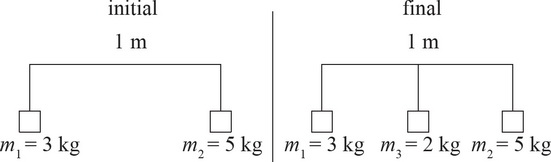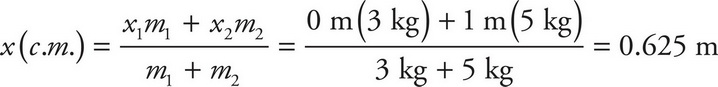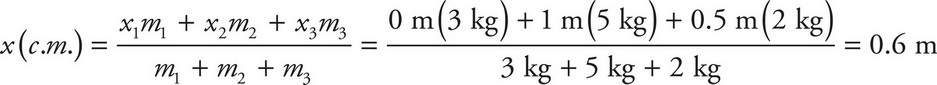# AP Physics 1 Question 448: Answer and Explanation

### Test Information

Question: 4486. Two masses are attached to a 1 m long massless bar. Mass 1 is 3 kg and is attached to the far left side of the bar. Mass 2 is 5 kg and is attached to the far right side of the bar. If a third mass that is 2 kg is added to the middle of the bar, how does the center of mass of the system change?

• A. The center of mass shifts to the left by 0.025 m.
• B. The center of mass shifts to the right by 0.025 m.
• C. The center of mass shifts to the left by 0.075 m.
• D. The center of mass shifts to the right by 0.075 m.

Explanation:

A

Start by calculating the center of mass for the system with two masses:Next, calculate the new center of the mass of the system with the addition of the third mass:The center of mass of the system has shifted to the left by 0.025 m.